# Information theory: the noisy binary symmetric channel¶

Florent Leclercq,
Imperial Centre for Inference and Cosmology, Imperial College London,
[email protected]

In :
import numpy as np
from scipy.special import binom
from matplotlib import pyplot as plt
from PIL import Image
np.random.seed(123456)
%matplotlib inline
plt.rcParams.update({'lines.linewidth': 2})
plt.rcParams.update({'font.size': 16})
plt.rcParams.update({'axes.titlesize': 16})
plt.rcParams.update({'axes.labelsize': 16})
plt.rcParams.update({'xtick.labelsize': 16})
plt.rcParams.update({'ytick.labelsize': 16})
plt.rcParams.update({'legend.fontsize': 16})
plt.rcParams.update({'figure.titlesize': 16})


## The signal¶

In :
# Open the image and convert it to an array of black/white pixels
im_data = Image.open('data/correlation.png')
im_grey = im_data.convert('L')
signal = np.array(im_grey, dtype=np.int)
signal[np.where(signal>1)]=1

In :
plt.figure(figsize=(8,4))
plt.axis('off')
plt.imshow(signal,cmap="Greys_r",interpolation=None)
plt.title("signal")
plt.show()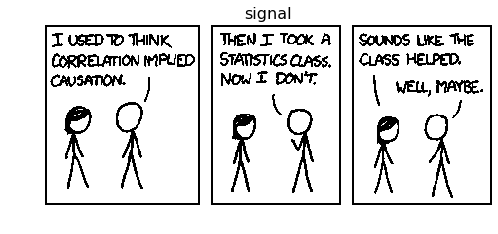## The noisy binary symmetric channel¶

The channel flips bits with a probability $f$: the noise level.

In :
def noisy_channel(transmitted,noise_level):

In :
noise_level=0.1

In :
sent=np.array((1,0,1,1,1,0,1,0,1,1,0,1,0,1,0,0,1,0,0,1,0,0,0))
print("sent :\t\t"+str(sent))

sent :		[1 0 1 1 1 0 1 0 1 1 0 1 0 1 0 0 1 0 0 1 0 0 0]
received :	[1 0 1 1 1 0 1 0 1 1 0 1 0 1 0 0 1 0 0 1 0 0 0]

In :
noisy_signal=noisy_channel(signal,noise_level)

In :
plt.figure(figsize=(8,4))
plt.axis('off')
plt.imshow(noisy_signal,cmap="Greys_r",interpolation=None)
plt.title("noisy signal")
plt.show()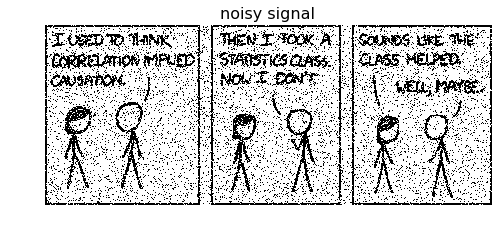## The R3 code¶

The R3 code is one of the simplest algorithms: the encoder just repeats the signal 3 times. The decoder uses a "majority vote" to decide the value of each bit of the decoded signal.

In :
def R3_encoder(signal):
m1=np.copy(signal)
m2=np.copy(signal)
m3=np.copy(signal)
return np.array((m1,m2,m3))

return decoded

In :
transmitted=R3_encoder(signal)

In :
plt.figure(figsize=(8,4))
plt.axis('off')
plt.imshow(transmitted,cmap="Greys_r",interpolation=None)
plt.title("transmitted (1st)")
plt.show()
plt.figure(figsize=(8,4))
plt.axis('off')
plt.imshow(transmitted,cmap="Greys_r",interpolation=None)
plt.title("transmitted (2nd)")
plt.show()
plt.figure(figsize=(8,4))
plt.axis('off')
plt.imshow(transmitted,cmap="Greys_r",interpolation=None)
plt.title("transmitted (3rd)")
plt.show()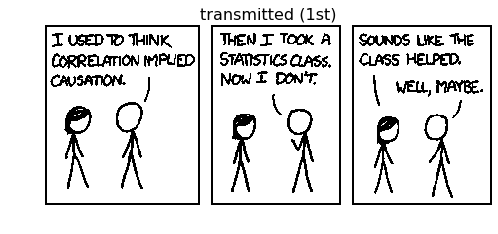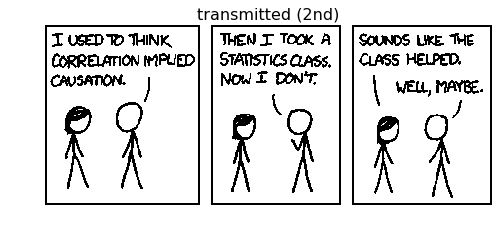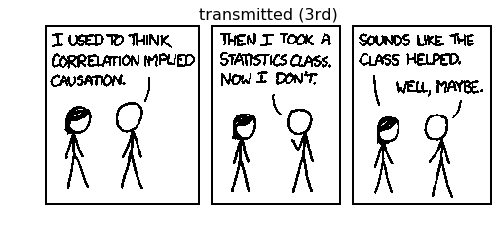In :
received=noisy_channel(transmitted,noise_level)

In :
plt.figure(figsize=(8,4))
plt.axis('off')
plt.show()
plt.figure(figsize=(8,4))
plt.axis('off')
plt.show()
plt.figure(figsize=(8,4))
plt.axis('off')
plt.show()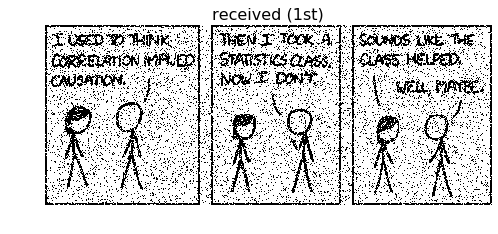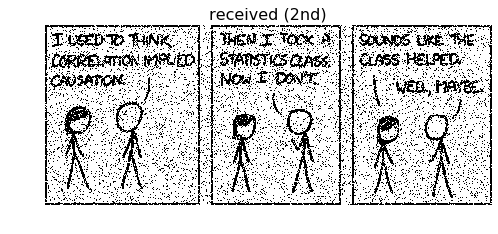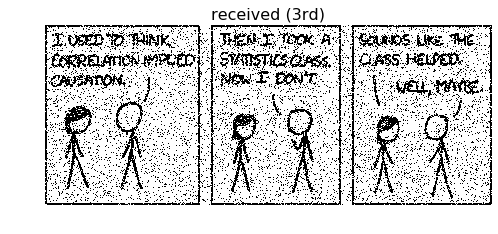In :
decoded=R3_decoder(received)

In :
plt.figure(figsize=(8,4))
plt.axis('off')
plt.imshow(decoded,cmap="Greys_r",interpolation=None)
plt.title("decoded")
plt.show()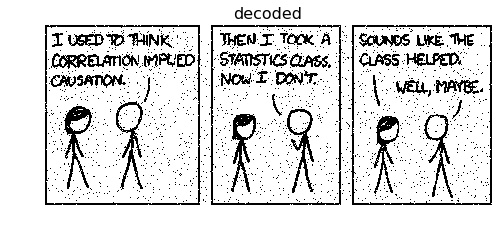In :
plt.figure(figsize=(8,4))
plt.axis('off')
plt.imshow(decoded-signal,cmap="bwr_r",interpolation=None)
plt.imshow(signal,cmap="Greys_r",alpha=0.2,interpolation=None)
plt.title("uncorrected errors")
plt.show()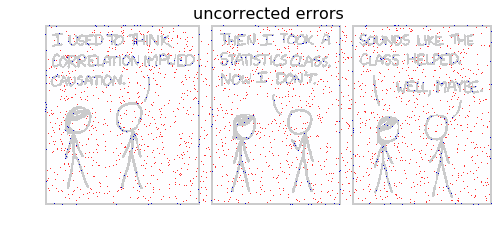Uncorrected errors are uncorrelated with the R3 code.

## The (7,4) Hamming code¶

The (7,4) Hamming code transmits the information by blocs of 4 bits $s_1$, $s_2$, $s_3$, $s_4$. For each block of 4 bits, 3 parity-checks bits $t_5$, $t_6$ and $t_7$ are also transmitted. The syndrome matrix allows to decode.

In :
Hamming_matrix=np.array([[1,0,0,0],[0,1,0,0],[0,0,1,0],[0,0,0,1],[1,1,1,0],[0,1,1,1],[1,0,1,1]])
syndrome_matrix=np.array([[1,1,1,0,1,0,0],[0,1,1,1,0,1,0],[1,0,1,1,0,0,1]])

# This function splits the full signal into 4-bit blocks, adding dummy bits at the end if necessary
def split_signal_4bits_blocks(signal):
split=signal.reshape(signal.shape*signal.shape)
N_dummy_bits=-len(split)%4
split=np.concatenate((split,np.zeros(N_dummy_bits)))
return np.split(split,len(split)/4)

# This is the Hamming encoder: transmitted = Hamming_matrix.dot(signal) for each 4-bit block
def Hamming_encoder(signal):
split_signal=split_signal_4bits_blocks(signal)
transmitted=np.zeros((len(split_signal),7),dtype=np.int)
for i in range(len(split_signal)):
signal_block=split_signal[i]
transmitted_block=Hamming_matrix.dot(signal_block)
transmitted[i]=transmitted_block
return np.mod(transmitted,2)

# This function computes the syndrome: syndrome = syndrome_matrix.dot(received) for each 7-bit block

# This function corrects the error in a received block, given its syndrome
if np.array_equal(syndrom,np.array((0,0,1))):
corrected_block+=1
elif np.array_equal(syndrom,np.array((0,1,0))):
corrected_block+=1
elif np.array_equal(syndrom,np.array((0,1,1))):
corrected_block+=1
elif np.array_equal(syndrom,np.array((1,0,0))):
corrected_block+=1
elif np.array_equal(syndrom,np.array((1,0,1))):
corrected_block+=1
elif np.array_equal(syndrom,np.array((1,1,0))):
corrected_block+=1
elif np.array_equal(syndrom,np.array((1,1,1))):
corrected_block+=1
return np.mod(corrected_block,2)

# This function translates corrected 7-bits blocks into 4-bit decoded blocks and removes dummy bits at the end
def reshape_corrected_7bits_blocks(corrected,signal_shape):
decoded=np.zeros((corrected.shape,4))

# for each 7-bits block, keep only the 4 decoded bits
for i in range(corrected.shape):
corrected_block=corrected[i]
decoded[i]=np.array((corrected_block,corrected_block,corrected_block,corrected_block)
,dtype=np.int)
decoded=decoded.flatten()
signal_length=signal_shape*signal_shape

# remove dummy bits at the end if necessary
N_dummy_bits=-signal_length%4
decoded=decoded[:-N_dummy_bits]
decoded=decoded.reshape(signal_shape,signal_shape)
return decoded

def reshape_parity_7bits_blocks(corrected,signal_shape):
parity=np.zeros((corrected.shape,3))

# for each 7-bits block, keep only the 4 decoded bits
for i in range(corrected.shape):
corrected_block=corrected[i]
parity[i]=np.array((corrected_block,corrected_block,corrected_block),dtype=np.int)

# reshape the array of parity bits to plot it as an image. dimensions are arbitrary here
parity=parity.flatten()
j=1
d=1
while(j<np.sqrt(len(parity))):
if len(parity) % j == 0:
d=j
j+=1

parity=parity.reshape(d,-1)
return parity

# This is the Hamming decoder
corrected[i]=corrected_block
return reshape_corrected_7bits_blocks(corrected,signal_shape)

In :
transmitted=Hamming_encoder(signal)
transmitted_parity=reshape_parity_7bits_blocks(transmitted,signal.shape)

In :
plt.figure(figsize=(8,4))
plt.axis('off')
plt.imshow(signal,cmap="Greys_r",interpolation=None)
plt.title("transmitted image")
plt.show()
plt.figure(figsize=(8,4))
plt.axis('off')
plt.imshow(transmitted_parity,cmap="Greys_r",interpolation=None)
plt.title("transmitted parity bits")
plt.show()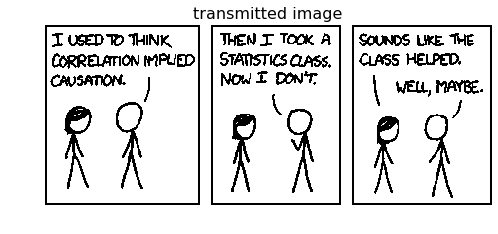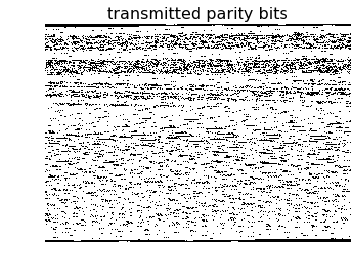In :
received=noisy_channel(transmitted,noise_level)

In :
plt.figure(figsize=(8,4))
plt.axis('off')
plt.figure(figsize=(8,4))
plt.axis('off')
plt.show()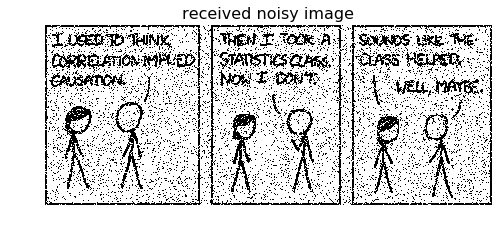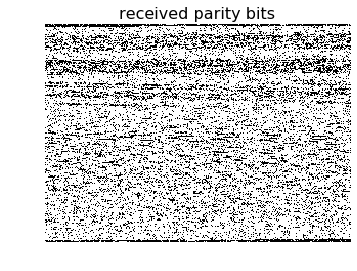In :
decoded=Hamming_decoder(received,signal.shape)

In :
plt.figure(figsize=(8,4))
plt.axis('off')
plt.imshow(decoded,cmap="Greys_r",interpolation=None)
plt.title("decoded")
plt.show()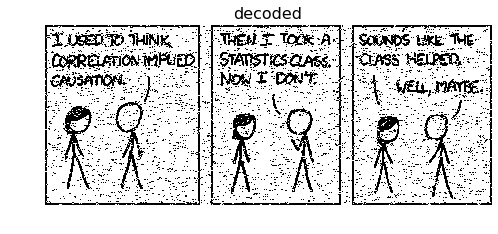In :
plt.figure(figsize=(8,4))
plt.axis('off')
plt.imshow(decoded-signal,cmap="bwr_r",interpolation=None)
plt.imshow(signal,cmap="Greys_r",alpha=0.2,interpolation=None)
plt.title("uncorrected errors")
plt.show()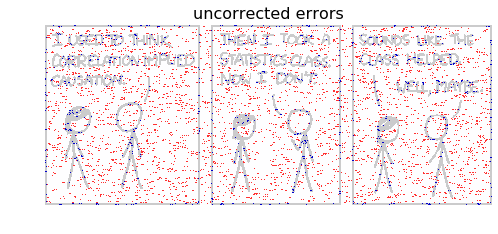There are more errors with the (7,4) Hamming code than with the R3 code, which is unsurprising since the rate of information transfer is $R = \frac{4}{7} > \frac{1}{3}$. Uncorrected errors are correlated, they happen preferentially in blocks of 4 consecutive bits.

## Shannon's noisy channel coding theorem¶

Rate of information transfer $R$ and probability of error $p_\mathrm{b}$ of repetition codes: for odd $N$: \begin{equation} R=\frac{1}{N} \end{equation} \begin{equation} p_\mathrm{b}=\sum_{(N+1)/2}^{N} \binom{N}{n} \, f^n (1-f)^{N-n} \end{equation} (MacKay 2003, equation (1.24))

In :
R=np.array([1./N for N in range(1,62,2)])
p=np.array([np.sum(np.array([binom(N,n)*noise_level**n*(1-noise_level)**(N-n)
for n in range((N+1)//2,N)])) for N in range(1,62,2)])
p=noise_level


Binary entropy function: \begin{equation} H_2(x) \equiv x \log \frac{1}{x} + (1-x)\log \frac{1}{1-x} \end{equation} Capacity of the noisy channel: \begin{equation} C(f) \equiv 1 - H_2(f) \end{equation} Shannon limit: \begin{equation} R=\frac{C(f)}{1-H_2(f)} \end{equation}

In :
def H2(f):
return f*np.log2(1./f) + (1-f)*np.log2(1./(1-f))
def capacity(noise_level):
return 1.-H2(noise_level)
def shannon_limit(p,noise_level):
return capacity(noise_level)/(1-H2(p))

In :
fig = plt.figure(figsize=(10,10))
ax.set_xlim(0,1)
ax.set_xlabel("$R$")
ax.set_xticks([0.0, 0.2, 0.4, 0.6, 0.8, 1.0, capacity(noise_level)])
ax.set_xticklabels(["0.0", "0.2", "0.4", "0.6", "0.8", "1.0", "$C(f)$"])
ax.set_yscale('log')
ax.set_ylim([1e-15,1e0])
ax.set_ylabel("$p_\mathrm{b}$")
ax.scatter(R,p,s=60,marker='.',color='blue',label="repetition codes")
ax.scatter([4./7.],[7e-2],s=60,marker='.',color='red')
p_shannon=np.linspace(noise_level,1e-15,1000)
ax.plot(shannon_limit(p_shannon,noise_level),p_shannon,color='black',lw=2,label="Shannon limit")
ax.fill_betweenx(p_shannon,shannon_limit(p_shannon,noise_level),np.ones_like(p_shannon),
facecolor='grey',alpha=0.3, linewidth=0.)
ax.set_title("Error probability versus rate - Shannon's theorem")
ax.text(0.333, 7e-3, '$R_3$', fontsize=16)
ax.text(0.965, 2e-2, '$R_1$', fontsize=16)
ax.text(0.58, 1e-1, '$H(7,4)$', fontsize=16)
ax.text(0.2, 1e-13, 'achievable', fontsize=16)
ax.text(0.6, 1e-13, 'not achievable', fontsize=16)
plt.legend(frameon=False,loc='center left',bbox_to_anchor=(1, 0.5))
plt.show()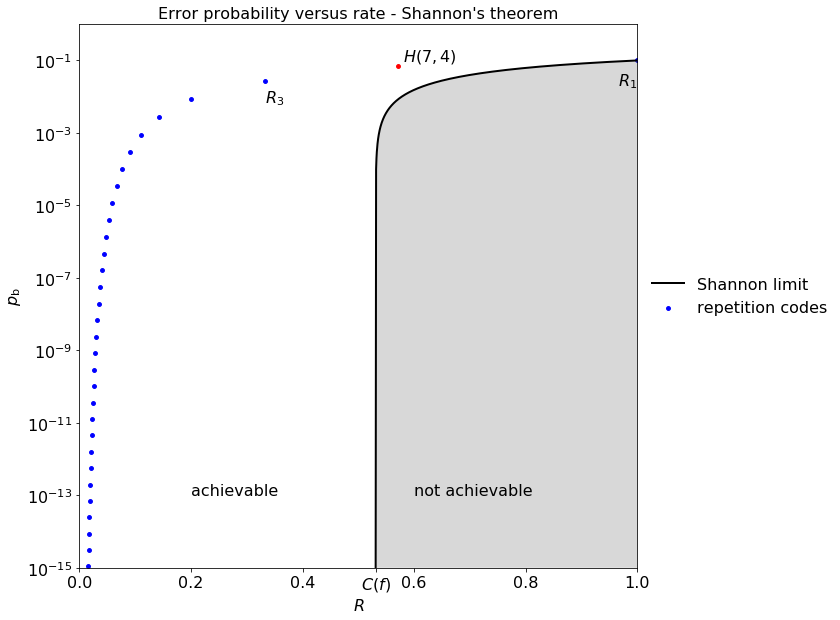Shannon's noisy-channel coding theorem: rates above the Shannon limit are achievable, rates below the Shannon limit are not achievable.

Rates up to $R=C(f)$ are achievable with arbitrarily small $p_\mathrm{b}$. The boundary between achievable and non-achievable points meets the $R$ axis at a non-zero value $R=C(f)$.